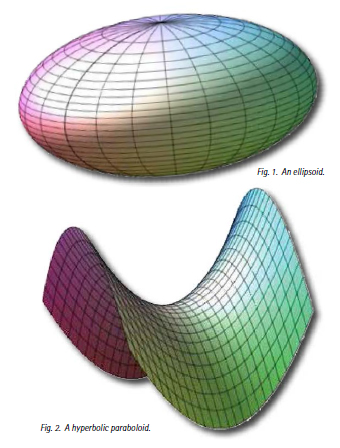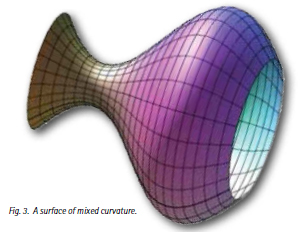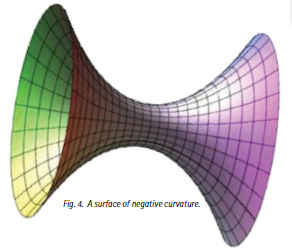THE SINGAPORE MAGAZINE OF RESEARCH, TECHNOLOGY AND EDUCATION About Innovation
 COVER STORY: Curvature and Hyperbolicity Properties of Certain Moduli Spaces Modifying the Weil-Petersson Geometry of Certain Moduli Spaces to Establish their Kobayashi Hyperbolicity by To Wing-Keungn the study of geometry, mathematicians are interested in how certain geometrical objects curve or osculate, and the curvature of a geometrical object is a measure of how the geometrical object is curving at its various points. In the familiar case of two-dimensional surfaces in the three-dimensional Euclidean space, the curvature at a point of the surface is a number which may be positive, zero or negative. When the surface is a plane (which, of course, does not curve at all), the curvature is zero everywhere on the plane. By contrast, an ellipsoid (see Figure 1) and a horse saddle (or more formally, a hyperbolic paraboloid; see Figure 2) do curve a lot. Furthermore, the ellipsoid and the horse saddle actually curve in different ways. To visualize this, one may take any point on the ellipsoid, and consider the tangent plane touching the ellipsoid at that point. Then one easily sees that the whole ellipsoid lies on one side of the tangent plane. On the other hand, when one considers the tangent plane touching the horse saddle at a given point, one observes that there are points of the horse saddle lying on each of the two sides of the tangent plane. The standard convention is that the ellipsoid is of positive curvature at each of its points, while the horse saddle is everywhere of negative curvature. An important class of higher dimensional geometrical objects are known as manifolds. Roughly speaking, for any positive integer n, an n-dimensional manifold is a geometrical object which locally looks like a piece of the n-dimensional Euclidean space. Ellipsoids and horse saddles are examples of two-dimensional manifolds. The notion of curvature has also been generalized and is applicable to manifolds.Recall also that complex numbers are numbers of the form x+iy, where x and y are real numbers and i denotes a square root of -1 in the sense that i2=-1. As such, the set of complex numbers are represented by points in the Argand diagram, which is simply the coordinate plane or the two-dimensional Euclidean plane. Explicitly, the complex number x+iy corresponds to the point (x,y). As a consequence, one may multiply two points in the Euclidean plane, since one can multiply two complex numbers! The Euclidean plane endowed with this additional multiplicative structure (or more formally, complex structure) is simply called the complex Euclidean plane. For any positive integer n, the n-dimensional complex Euclidean space is simply the n-fold Cartesian product of the complex Euclidean plane. More generally, an n-dimensional complex manifold is simply a geometrical object which locally looks like a piece of the n-dimensional complex Euclidean space. In particular, an n-dimensional complex manifold is also a 2n-dimensional (real) manifold. We are concerned with interaction between geometric and analytic properties of complex manifolds. Complex manifolds arise naturally in and are deeply connected to other branches of mathematics, including hyperbolic geometry, algebraic geometry, symplectic geometry, number theory and mathematical physics such as string theory. In this article, we will focus on those complex manifolds that arise as moduli spaces (or equivalently parameter spaces) that parameterize certain geometrical objects.One-dimensional complex manifolds are also known as Riemann surfaces. By the uniformization theorem, the universal cover of a Riemann surface is either the complex projective plane, the complex Euclidean plane C, or the complex hyperbolic plane. Geometrically the complex hyperbolic plane is endowed with the complete hyperbolic metric of constant negative curvature. Analytically, the complex hyperbolic plane can be identified with the unit disc ={zC:|z|<1} in the complex Euclidean plane. Then it follows from Liouvilles Theorem in complex analysis that any holomorphic map from C to the complex hyperbolic plane is necessarily a constant map. This notion has been generalized to the higher dimensional case, and one says that a complex manifold M is Brody hyperbolic if any holomorphic map f: CM must be a constant map. We remark that the complex projective plane and the complex Euclidean plane are not Brody hyperbolic. Generally speaking, hyperbolicity refers to certain property of complex manifolds possessed by the complex hyperbolic plane but not possessed by the complex projective plane or the complex Euclidean plane. Now we recall the moduli space Mg of compact Riemann surfaces X of genus g 2 (which form an important subset of the set of all Riemann surfaces), which roughly speaking, parameterizes the complex structures on the underlying surface of X. Strictly speaking, Mg is not a complex manifold, but it admits a smooth covering which is a (3g-3)-dimensional complex manifold known as the Teichmüller space Tg. The hyperbolicity properties of Tg can be studied from a differential geometric perspective. For this, we recall that Tg is endowed with a distinguished metric called the Weil-Petersson metric (roughly speaking, once a manifold has been endowed with a metric, we can talk about the distance between any two points in the manifold, and we can also talk about the geometry of the manifold associated to the metric, etc.). By the works of Ahlfors (, ), Royden  and Wolpert , one knows that Tg (with the geometry associated to the Weil-Petersson metric) is everywhere of negative curvature. In fact, their results imply that Tg possesses certain hyperbolicity property known as Kobayashi hyperbolicity, which is a stronger property than Brody hyperbolicity (see ,  for more detailed discussion).It is interesting and natural to ask whether the property of Kobayashi hyperbolicity is possessed by the moduli spaces of n-dimensional complex manifolds when n 2. The first breakthrough along this direction was made by Siu  who computed the curvature tensor of the Weil-Petersson metric on the moduli spaces of an important class of complex manifolds known as canonically polarized manifolds (Aubin  and Yau  showed that every canonically polarized manifold admits a nice metric known as the Kähler-Einstein metric). It turns out that the expression of the curvature of the Weil-Petersson metric contains negative terms as well as a positive term (the positive term only appears when n 2), and as such, one cannot decide conclusively the question raised in the beginning of this paragraph, even though an expression of the curvature tensor is already available (see also ,  for related works in this direction). There is also no known example which can give a negative answer to this open folklore question, which has been around since the 1980s. Recently the author, in collaboration with Sai-Kee Yeung of Purdue University, made some progress in this direction (see ), which we describe briefly here. Recall that when n 2, the curvature tensor of the Weil-Petersson metric contains some good terms which are negative as well as a bad term which is positive. In a somewhat simplified picture, our approach is to modify the Weil-Petersson metric by this bad term. The curvature expression of the modified metric will again contain good terms (which are negative) as well as a bad positive term. The process is repeated, and eventually for any effectively parameterized family of canonically polarized manifolds over a base complex manifold, one obtains a metric on the base complex manifold whose curvature is everywhere negative. Roughly speaking, we have modified the geometry of the base complex manifold which initially contains ellipsoidal points as well as saddle-like points (see Figure 3) so that the base complex manifold with the new geometry contains only saddle-like points (see Figure 4). In fact, our result implies that the base complex manifold is necessarily Kobayashi hyperbolic. We remark that Viehweg and Zuo  showed earlier, using algebraic geometric methods, that such base complex manifold is Brody hyperbolic. Currently the author and his collaborator are investigating how the approach in  may be adapted to study other moduli spaces.